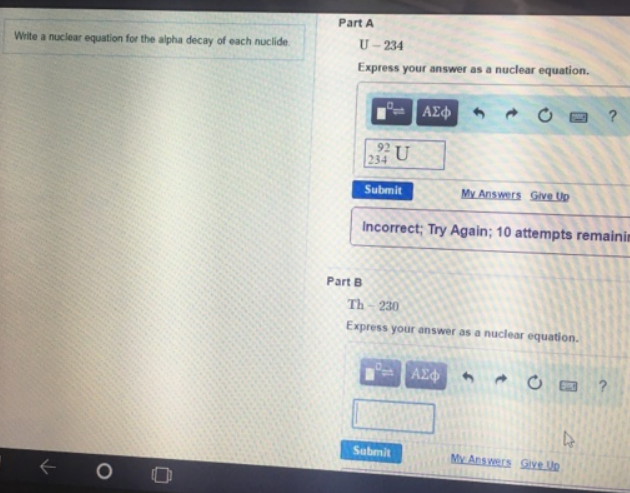# Problem: Write a nuclear equation for the alpha decay of each nuclide.U-234Th-230Express your answer as a nuclear equation.

###### FREE Expert Solution
89% (151 ratings)###### Problem Details

Write a nuclear equation for the alpha decay of each nuclide.

U-234
Th-230